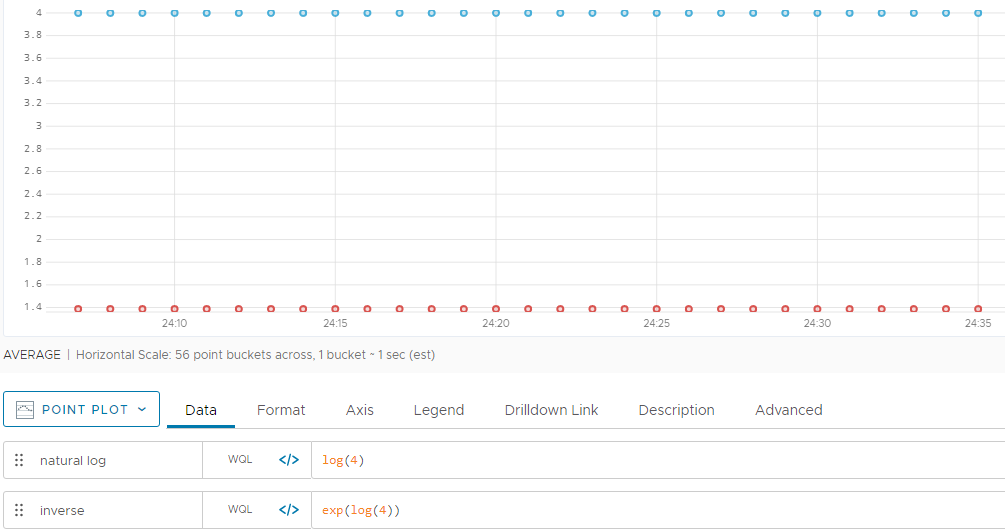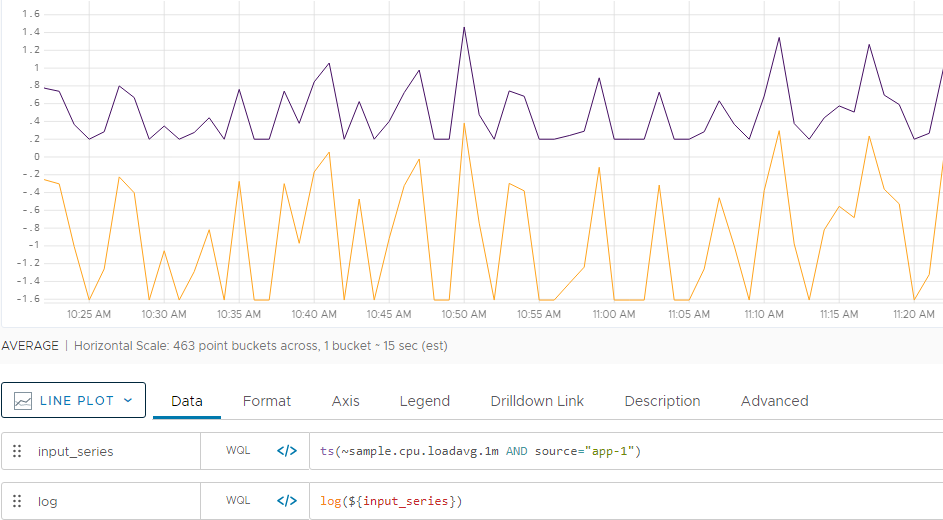Reference to the log() function

## Summary

``````log(<tsExpression>)
``````

Returns the natural logarithm of each data value described by the expression.

## Parameters

ParameterDescription
tsExpression Expression describing a constant or a time series of values to take the natural logarithm of.

## Description

The `log()` function produces a time series in which each data value is equal to the natural logarithm (log base e) of the value or values specified by the expression. The natural logarithm is the power to which you need to raise e to obtain the specified value. In mathematics, `loge` can be written `ln`.

• If `tsExpression` describes a constant value `N`, then `log()` returns a continuous series where every data value is `loge N`.
• If `tsExpression` describes one or more time series, then `log()` returns a new time series for each input time series. Each value in a new time series is calculated as `loge V`, where `V` is the value of the corresponding point in the input time series.

The inverse of this function is `exp()`.

By default, the chart legend displays a rounded version of the result. You can cause the legend to display the result with more precision by holding down the shift key when you hover over the time series.

## Examples

In this example, we see the result (in red) of using `log(4)` to find the power of e that equals 4. We also see the result (in blue) of obtaining the inverse by applying the `exp()` function to the result of `log(4)`.Here we see the result (in orange) of using `log()` to take the natural log of a time series (in purple).